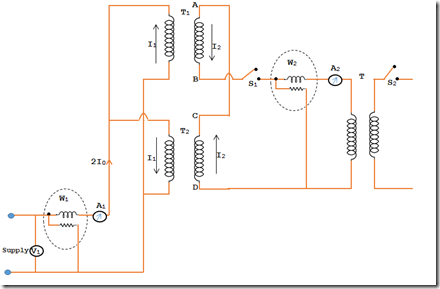# Sumpner Test or Back to Back Test on Transformer

This test is conducted instantaneously on two similar transformers and so the transformers temperature rise, efficiency and voltage regulations. The advantage of conducting this test is very economical of power, even when the transformers are tested at full load conditions. The total losses of both the transformers are equal to the power required to conduct this test. It might be noted to carry out this test that the two transformer needs to be identical.

### Circuit:The figure above shows that the connection diagram of back to back test on two similar transformers named T1 and T2. The two transformers primary windings are parallel connected across the rated supply voltage V1 and the secondary windings of the transformers are connected opposition in phase. Hence the circulating current formed in the loop of secondary’s will be zero because EMF induced in the transformers are equal and in opposition. There is an auxiliary low voltage in the transformer which can be varied to provide an adjustable voltage and so current in secondary circuit loop. The volt-meter V1, amp-meter A1 and watt-meter W1 are connected in the primary side (input) of the transformer. An amp-meter A2 and watt-meter W2 are connected in secondary (output side) of the transformer.Methods of Transformer Cooling

### Working:

The transformer secondary sides are in phase opposition. Then switch S2 is open and switch S1 is closed, thus the circulating current in the transformer secondary circuit loop is zero (i.e. I2=0). This is due to EMF induced in the secondary’s are in equal and opposition. This circumstance is just like an open circuit test. Hence the current drawn from the source is 2I0. The wattmeter reading W1 and the core loss of both the transformers are equal.

I0= No load current of every transformer
W1 = Core losses of both the transformers.

Now switch S2 also closed and the voltage at the output of the regulating transformer is varied until the full load current I2 drift in the secondary circuit loop. The secondary full load current will cause full load current I1 in the primary circuit. The full load current I1 will circulates in the primary winding alone. The two transformers full load copper losses is equal wattmeter W2 reading since the full load current circulating through the primary winding and secondary windings.

W2 = Transformer full load copper losses
W2+W1 = Total losses of the two transformers at full load.

#### During this test the following point may be noted:

• The wattmeter’s W1 & W2 gives the core losses and copper losses at full load of the two transformers respectively. Hence the total losses of two transformers are equal to the power required to conduct this test.
• The total iron losses and copper losses at full load are occurring even though the transformers are not supplying any load.
• There are two voltage supply; one is transformer regulating voltage and the other is supply voltage and in between these voltages no interference. The source voltage provides 2I0 while the transformer regulating voltage provides I2 and thus I1=KI2.Voltage consideration in Designing of Transformer

• Little much of power is required to conduct this test
• Under full load conditions transformers can be test using this test.
• Simultaneously full load copper losses and iron losses are measured
• The secondary current I2 can be varied at any value of the current. Hence we can determine the copper losses at full load condition or at any load.
• The transformer temperature increase can be noted.This post first appeared on My Tech Info, please read the originial post: here

# Share the post

Sumpner Test or Back to Back Test on Transformer

×